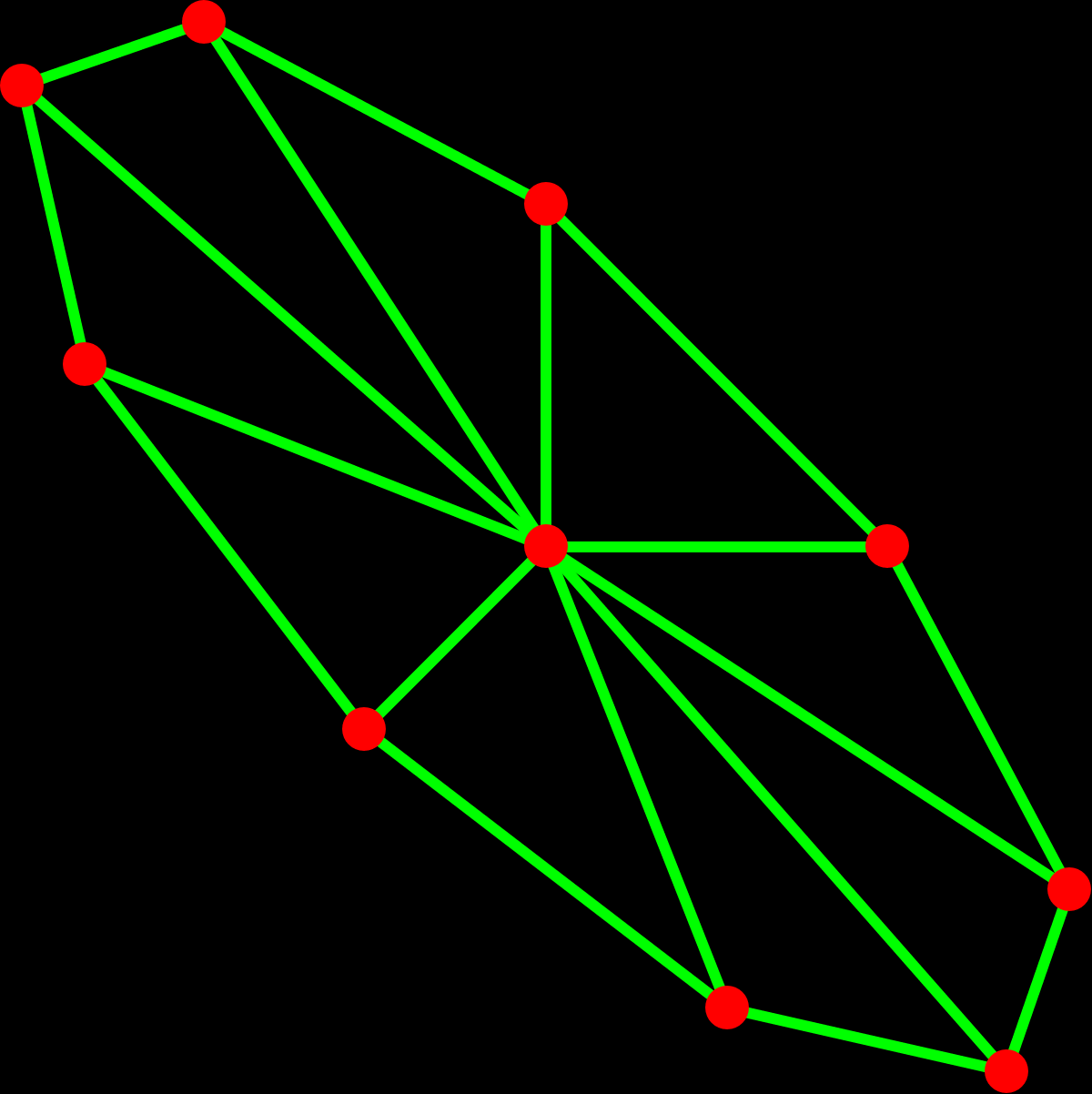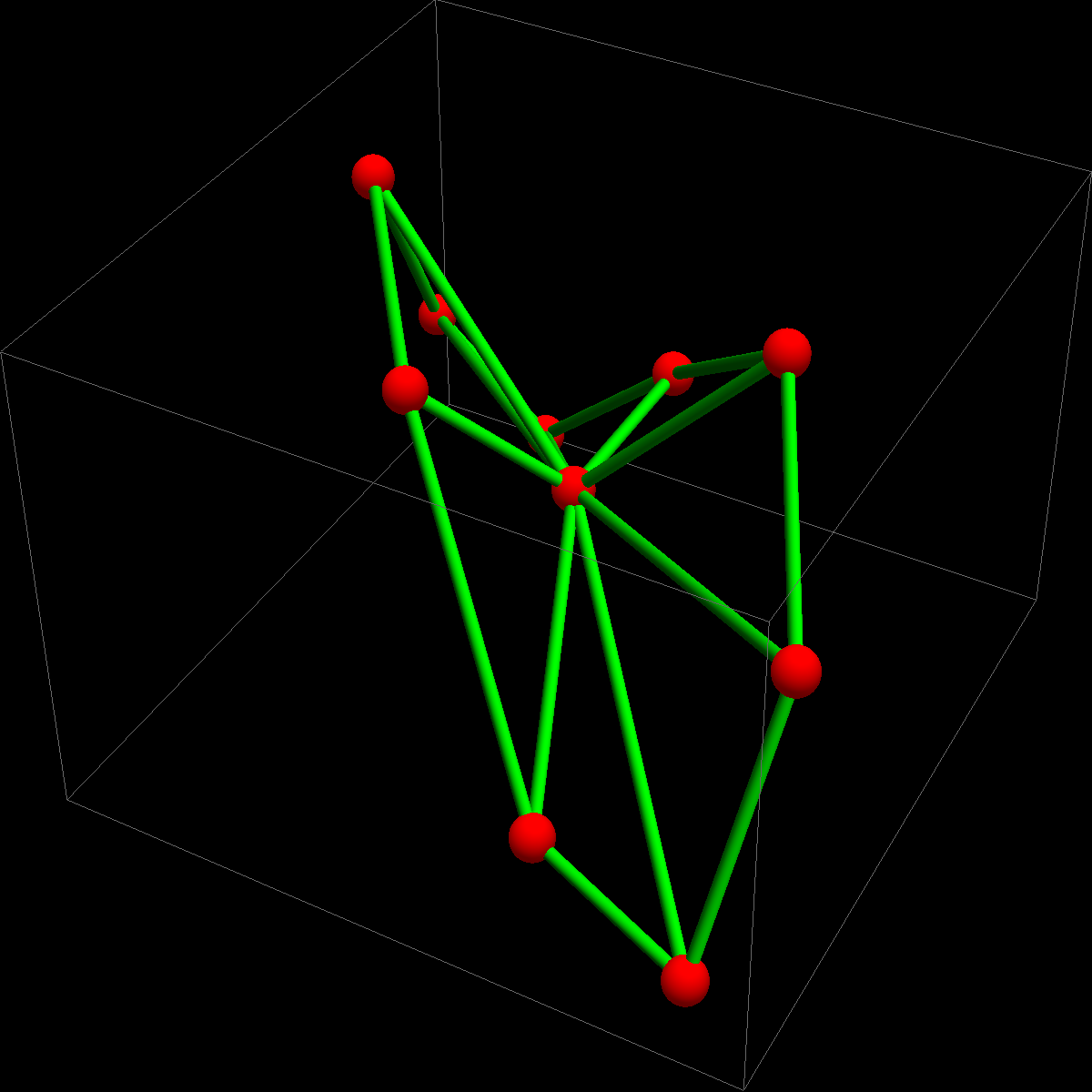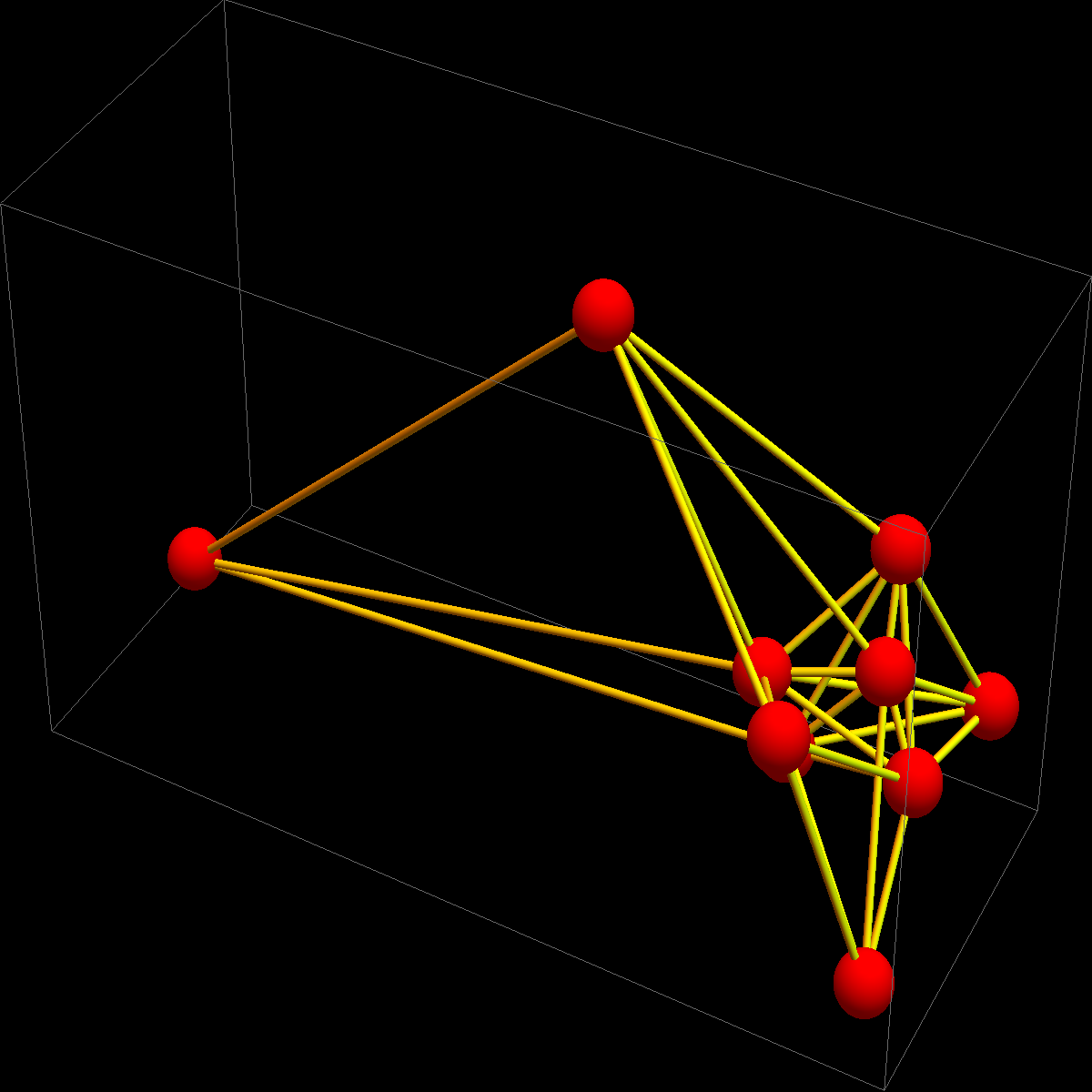M A T H 2 1 B
Mathematics Math21b Spring 2016
Linear Algebra and Differential Equations
Exhibit: Spectral embedding
Office: SciCtr 432

## Spectral embedding

A student mentioned that in a LS1b lecture of Pardis Sabeti of April 21, 2016 (called Microbiome, CRISPR and New Frontiers"), one of the slides featured an eigenvector picture. What is that. We try to explain the math.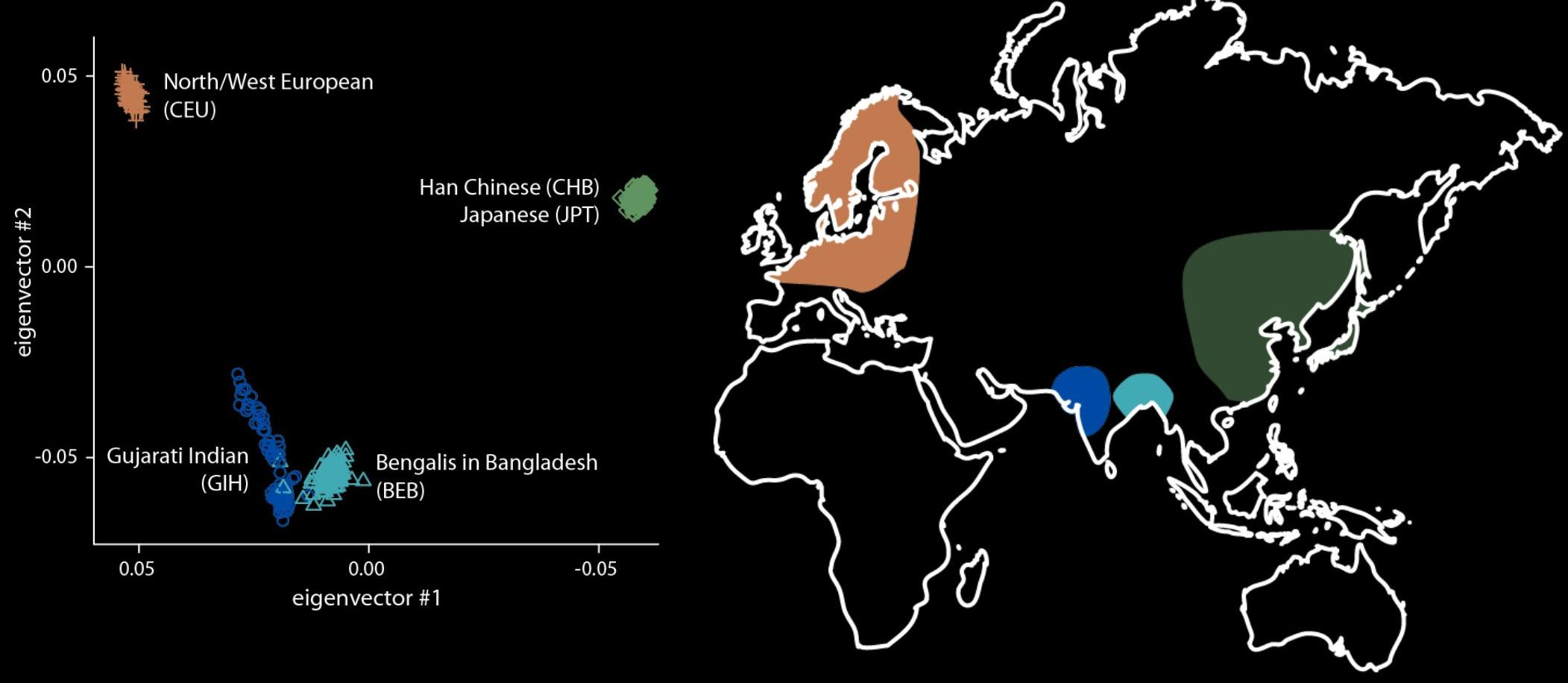What is a spectral embedding? The idea comes from linear algebra and graph theory. Let L be the Kirchhoff matrix of the graph (you have seen it in the mathematica project or in the final exam). This matrix always has the eigenvector v1 = [1,1,1...1]T. Now look at the eigenvectors v2 and v3.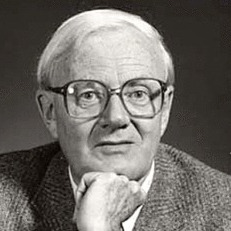The spectral embedding is to place the k'th node at the point in the plane which has the coordinates P = (v2(k), V3(k)). This method goes back to William Thomas Tutte and has been refined more and more, using also other, higher eigenfunctions. We can for example embed into three dimensional space placing the k'th node at P = (v2(k), V3(k), V4(k) ) Below we see two pictures, where a wheel graph with 10 vertices is embedded first in the plane than in space using the spectral embedding. The third picture is a random graph embedded in space.